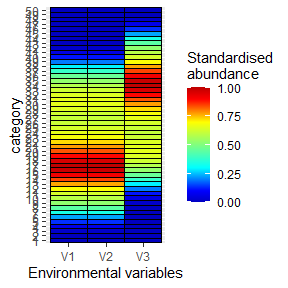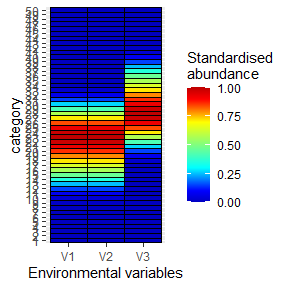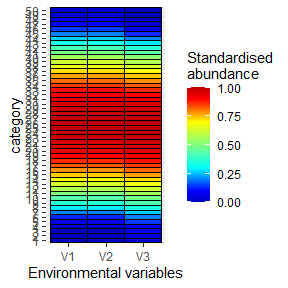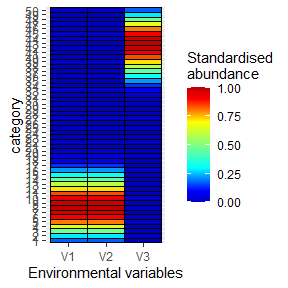# specieschrom

#### 2022-10-14

In this document, we describe how to use the R package specieschrom available on Github. The species chromatogram method only uses three main functions : chromato_env16.R, opti_eury_niche2_v2.R and combina_niche3.R. The following packages are required: abind, colorRamps, ggplot2, reshape2 and utils. Noted that the same procedure is applicable with Matlab (functions are also available on Github).

See Kléparski and Beaugrand 2022, Ecology and Evolution 12:e8830 (https://doi.org/10.1002/ece3.8830) for further details.

The package can be installed from Github with the devtools package:

devtools::install_github("loick-klpr/specieschrom")

### Function chromato_env16.R

The function chromato_env16.R estimates and displays the chromatogram of a given species. This function takes six arguments in the following order:

chromato_env16(z,y,alpha,m,k,order_smth)

With z a matrix with n samples by p environmental variables (i.e. the value of each environmental variable in each sample), y a vector with the abundance of a species in the n samples, alpha an integer corresponding to the number of category along each environmental variable, m an integer corresponding to the lowest number of samples needed in a category in order to have an estimation of the mean abundance, k an integer corresponding to the percentage of samples with the highest abundance values to use to estimate the mean abundance in a given category and order_smth an integer corresponding to the order of the simple moving average applied along each niche dimension. The simple moving average is applied to reduce the noise in the mean abundance sometimes observed in the chromatograms from a category to another.

chromato_env16.R used the functions nanmean4.R, which estimates the mean of the k% of the samples with the highest abundance and moymob1.R which applies the moving average.

### Example with simulated data:

Load the specieschrom R package and the datasets with the 14 pseudo-species abundances and the associated environmental variables.

library(specieschrom)

data("data_abundance")
data("environment")

The abundance dataset contains the abundance of 14 pseudo-species (columns PS1 to PS14) in 100 samples (lines). Pseudo-species were generated with a beta-niche. Noted that each pseudo-species has been duplicated (2 x 7 pseudo-species). The environment dataset contains the values of three fictive environmental variables (columns x1 to x3) in the 100 samples (lines).

Apply the function chromato_env16.R on the first pseudo-species to display its three dimensional niche. Here we used alpha=50 categories, m=1 sample at least in each category, k=5 and order_smth=2 :

sp_chrom_PS1<-chromato_env16(environment,data_abundance[,1],50,1,5,2)With the third pseudo-species:

sp_chrom_PS3<-chromato_env16(environment,data_abundance[,3],50,1,5,2)With the fifth pseudo-species:

sp_chrom_PS5<-chromato_env16(environment,data_abundance[,5],50,1,5,2)With the eighth pseudo-species:

sp_chrom_PS8<-chromato_env16(environment,data_abundance[,8],50,1,5,2)### Function opti_eury_niche2.R

The function opti_eury_niche2.R estimates the niche optimums and breadths of each species along each niche dimension, i.e. each environmental variable. The mean niche breadth is also estimated. This function takes six arguments in the following order :

opti_eury_niche2(sp_chr,Thres_T,z,y,k)

With sp_chr a three dimensional matrices with the species chromatograms (alpha category by p environmental variables by species), Thres_T the threshold of minimal abundance in a category for the niche breadth estimation, z a matrix with n samples by p environmental variables (i.e. the value of each environmental variable in each sample), y a matrix with the abundance of the species in the n samples and k the percentage of samples with the highest abundance used for the mean abundance estimation.

### Example with simulated data:

Combine the multiple species chromatograms along the third dimension with the function abind:

library(abind)
test_PS<-abind::abind(sp_chrom_PS1,sp_chrom_PS3,sp_chrom_PS5,sp_chrom_PS8,along=3)

The function opti_eury_niche2.R can then be applied, with a threshold of abundance Thres_T=0 and k=5 (as in chromato_env16.R):

opti_ampli_niche<-opti_eury_niche2(test_PS,0,environment,data_abundance[,c(1,3,5,8)],5)

The degree of euryoecy (i.e. niche breadth) of each pseudo-species along each dimension are stored in opti_ampli_niche$amplitudes, a table with in line the p environmental variables (here three) and in column the pseudo-species (here four, i.e. 1=PS1, 2=PS3, 3=PS5 and 4=PS8): opti_ampli_niche$amplitudes
##      [,1] [,2] [,3] [,4]
## [1,]   80   50   96   40
## [2,]   80   50   96   40
## [3,]   80   50   96   40

The mean degree of euryoecy of each pseudo-species is stored in opti_ampli_niche$mean_amplitudes: opti_ampli_niche$mean_amplitudes
##  80 50 96 40

The niche optimums for each species (i.e. four, in column) along each niche dimension (i.e. three, in line) are stored in opti_ampli_niche$optimums: opti_ampli_niche$optimums
##            [,1]       [,2]       [,3]      [,4]
## [1,]  8.0808081 11.3636364 12.6262626 3.7878788
## [2,] 12.9292929 18.1818182 20.2020202 6.0606061
## [3,]  0.6767677  0.5454545  0.4949495 0.8484848

### Function combina_niche3.R

The function combina_niche3.R estimates the index of niche overlapping (D) among species niche. It returns three matrices: combi_dim which contains the combination of environmental variables associated with the lowest degree a niche overlapping when 1 to p environmental dimensions are considered, sp_by_sp which contains the degree of niche overlapping species by species when all the environmental variables are considered and dim_alone which contains the lowest degree of niche overlapping when each environmental variable is considered alone. This function takes two arguments in the following order :

combina_niche3(sp_chr,Thres_T)

With sp_chr a three dimensional matrix with the species chromatograms (alpha category by p environmental variables by species) and Thres_T the threshold of minimal abundance in a category for the niche breadth estimation.

combina_niche3.R used the functions niche_difer_sp.R and niche_difer2.R.

### Example with simulated data:

Apply the function on the previous three-dimensional matrix test_PS:

Index_D_PS<-combina_niche3(test_PS,0)

In combi_dim the first column displays the number of dimensions considered simultaneously and columns 2 to 4 display the combinations of dimensions considered. The last column displays the index D associated with the combination of environmental dimensions. D=0% when species niches are fully different and D=100% when species niches are identical; the higher the number of dimensions, the lower the value of index D. Only the combinations of environmental variables that minimise values of index D are displayed :

Index_D_PS$combi_dim ## [,1] [,2] [,3] [,4] [,5] ## [1,] 1 1 NA NA 52.18957 ## [2,] 2 1 2 NA 30.82066 ## [3,] 3 1 2 3 19.71507 The matrix sp_by_sp contains the degree of niche overlapping species by species when all the environmental dimensions are considered : Index_D_PS$sp_by_sp
##      [,1]     [,2]     [,3]     [,4]
## [1,]   NA 24.41406 57.87037 8.813928
## [2,]   NA       NA 14.12851 7.891492
## [3,]   NA       NA       NA 5.172047
## [4,]   NA       NA       NA       NA

The matrix dim_alone contains the lowest degree of niche overlapping when each environmental variable is considered alone. Here, as each species has the same niche breadth along each dimension (because of the use of a beta-niche), the same degree of niche overlapping is observed along each dimension :

Index_D_PS\$dim_alone
##  52.18957 52.18957 52.18957